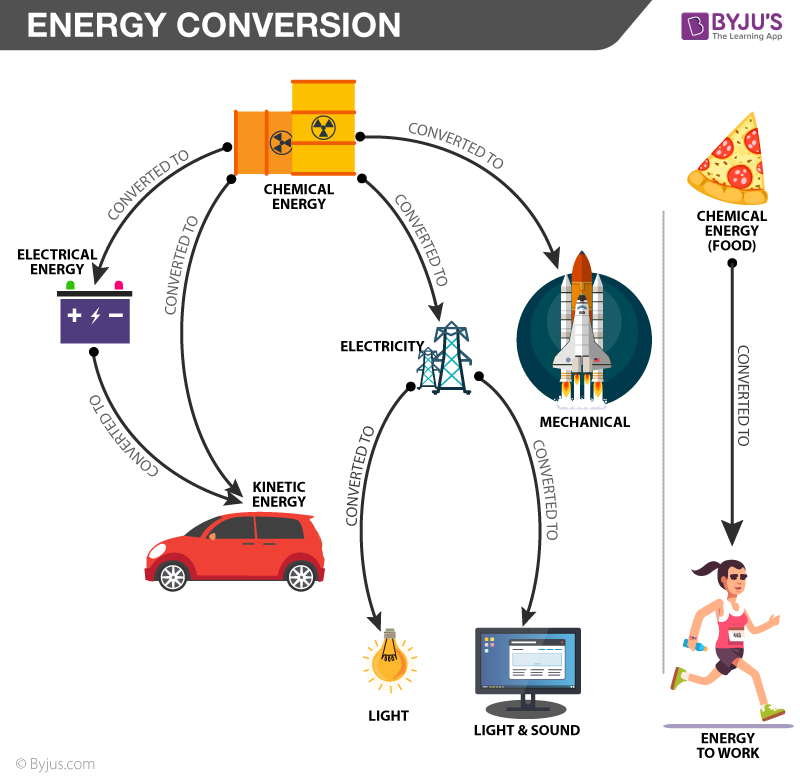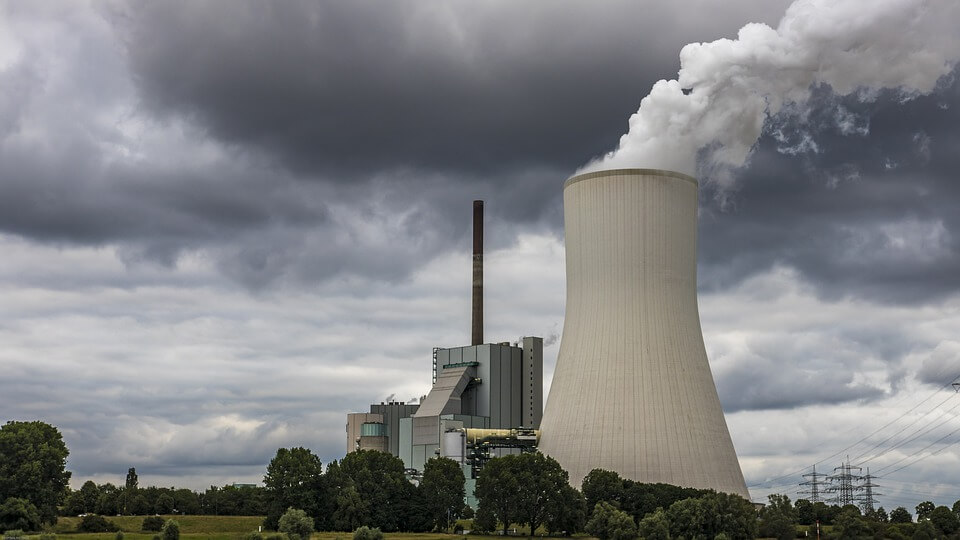Checkout JEE MAINS 2022 Question Paper Analysis : Checkout JEE MAINS 2022 Question Paper Analysis :

# Energy Conversion

Energy conversion, also termed as energy transformation, is the process of changing one form of energy into another. Energy conversion occurs everywhere and every minute of the day. There are numerous forms of energy like thermal energy, electrical energy, nuclear energy, electromagnetic energy, mechanical energy, chemical energy, sound energy, etc. On the other hand, the term Energy Transformation is used when energy changes forms from one form to another. Whether the energy is transferred or transformed, the total amount of energy doesn’t change and this is known as the Law of Conservation of Energy.

## The Law of Energy ConversionThe diagram shows how different forms of energy can be converted to another form of energy

Thermodynamics is the study of how energy gets converted from one form to another. Details on how the laws of thermodynamics apply to the conversion of energy are given below.

The first law of thermodynamics states that

Energy can neither be created nor destroyed, it can only be transformed from one form to another.

This is also known as the law of conservation of energy or the law of energy conversion. There are various types and forms of energy. Some examples of everyday energy conversions are provided below.

 Scenario Energy conversions involved Rubbing both hands together for warmth Kinetic Energy to Thermal Energy A falling object speeding up Gravitational Potential Energy to Kinetic Energy Using battery-powered torchlight In the battery: Chemical to Electrical Energy In the bulb: Electrical to Radiant Energy In Geothermal Power Plant Heat Energy to Electrical Energy In Thermocouple Heat Energy to Electrical Energy In Hydroelectric Dams Gravitational potential energy to Electric Energy In Electric Generator Kinetic energy / Mechanical work to Electric Energy In Windmills Wind Energy to Mechanical Energy or Electric Energy In OTEC Heat Energy to Electric Energy or Mechanical Energy Using Microphone Sound Energy to Electric Energy Photosynthesis in Plants Solar Energy to Chemical Energy In Piezoelectrics Strain to Electric Energy In Electric lamp Electric Energy to Heat and Light Energy Burning of wood Chemical energy to Heat and Light Energy In Fuel cells Chemical Energy to Electric Energy In steam engine The heat energy to Mechanical Energy In Electric heater Electric Energy to Heat

## Examples of Energy Conversion

Some examples of sets of energy conversion in machines are explained below.

### Coal-fired Power PlantIn coal-fired powerplants, the chemical energy in the coal after a series of transformations, gets converted into electrical energy

The sets of energy transformation inside a coal-fired power plant are given in the points below.

• During the process of combustion, the chemical energy in the coal gets converted into thermal energy.
• Then through the heat exchanger, the thermal energy of the exhaust gases gets converted into thermal energy of steam.
• After that in the turbine, the thermal energy of steam gets converted into mechanical energy.
• Finally, with the help of a generator, the mechanical energy gets converted into electrical energy.

### Conventional Automobile

The sets of energy transformation in a conventional automobile are given in the points below.

• Due to combustion, the chemical energy in the fuel gets converted into kinetic energy of the expanded gas.
• Then the kinetic energy of the expanding gas gets converted into linear piston movement.
• Then the linear piston movement gets converted into rotary crankshaft movement.
• The rotary movement of drive wheels gets converted into linear motion of the automobile.

## Energy Transfer vs Energy Transformation vs Energy Conservation

### What is Energy Transfer?

The movement of energy from one location to another.

### What is Energy Transformation?

The process of the changing of the energy from one type to another.

### What is Energy Conservation?

It means that the total amount of energy remains the same.

## Practice Questions

### Using the following diagram answer the following questions. Neglect the effect of resistance forces.• What happens to the sum of gravitational potential and kinetic energies when the object moves from point A to point D across the surface?

Answer: The total mechanical energy (i.e., the sum of the kinetic and potential energies) remains the same whenever there are no external or nonconservative forces (such as friction or air resistance) doing work.

• At what point will the object have a minimum gravitational potential energy?

Answer: The gravitational potential energy depends on the height. The potential energy is minimum when the height is minimum. At point B, the object will have minimum gravitational potential energy.

• At which point in the diagram is the object’s kinetic energy lesser than the object’s kinetic energy at C?

Answer: Since the total mechanical energy is conserved, kinetic energy will be greatest when the potential energy is smallest. Point B is the only point that is lower than point C. The reasoning would follow that point B is the point with the smallest PE, the greatest KE, and the greatest speed. Therefore, the object will have less kinetic energy at point C than at point B (only).

Stay tuned with BYJU’S to learn more interesting Physics topics like Ohm’s Law, Thermodynamics, Laws of Motion, etc.

## What is Kinetic Energy and Potential Energy?Test Your Knowledge On Energy Conversion!